﻿ Growth of Polynomials not Vanishing inside a Circle

### Growth of Polynomials not Vanishing inside a Circle

Gulshan SinghOPEN ACCESSPEER-REVIEWED

## Growth of Polynomials not Vanishing inside a Circle

Department of Mathematics Jammu and Kashmir State Government of Education India

### Abstractis a polynomial of degree n, having no zeros in |z| < 1, then it was proved by Aziz and Dawood, [J. Approx. Theory, 53 (1988), 155-162] that for R≥1,In this paper, we refine above result for the polynomials P(z) of degree n≥4.

• Singh, Gulshan. "Growth of Polynomials not Vanishing inside a Circle." Journal of Mathematical Sciences and Applications 2.2 (2014): 25-27.
• Singh, G. (2014). Growth of Polynomials not Vanishing inside a Circle. Journal of Mathematical Sciences and Applications, 2(2), 25-27.
• Singh, Gulshan. "Growth of Polynomials not Vanishing inside a Circle." Journal of Mathematical Sciences and Applications 2, no. 2 (2014): 25-27.

 Import into BibTeX Import into EndNote Import into RefMan Import into RefWorks

### 1. Introduction and Statement of Results

For a polynomial P(z) of degree n, it is well known and is a simple consequence of maximum modulus principle (see ) that for R≥1,(1)

with equality holding for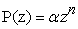, α being a complex number. Ankeny and Rivlin  proved that if P(z)≠ 0 in |z| < 1, then (1) can be replaced by(2)

Here equality holds for.

As a refinement of inequality (2), Aziz and Dawood  proved:

Theorem A If P(z) is a polynomial of degree n and P(z)≠0 in |z| < 1, then for R≥1,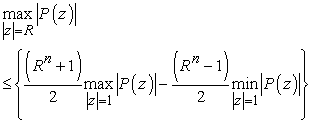(3)

The result is best possible and equality holds for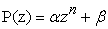, where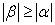.

In this paper, we prove the following result which is a refinement of Theorem A.

Theorem 1: Let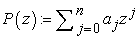be a polynomial of degree n≥4 having no zeros in |z| < 1, then for R≥1,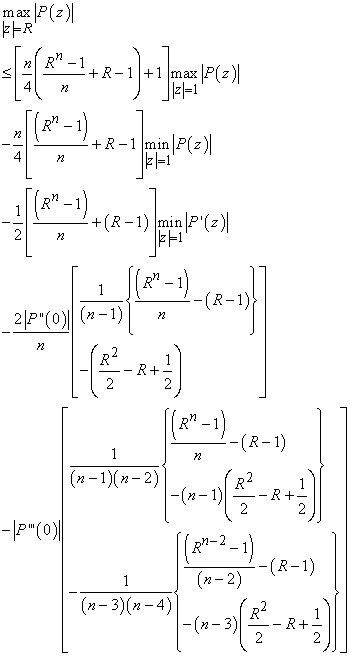(4)

if n>4, and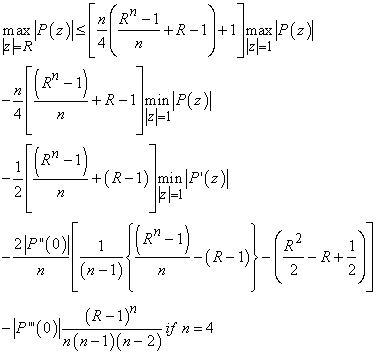(5)

### 2. Lemmas

For the proof of the theorem 1, we need the following lemmas. The first result was proved by Aziz and Dawood .

Lemma 1: If P(z) is a polynomial of degree n having no zeros in |z| < 1, then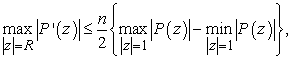The next result is a special case of a result due to Dewan, Singh and Mir  with k = μ = 1.

Lemma 2: Ifis a polynomial of degree n ≥ 3 having no zeros in |z| < 1, then for R ≥ 1,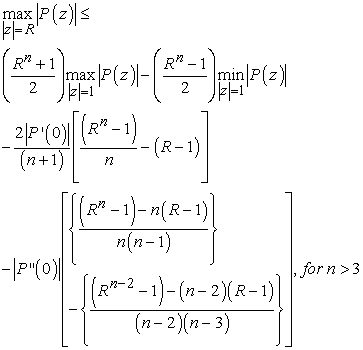(6)(7)

### 3. Proof of the Theorem 1

For each θ, 0 ≤θ < 2π and for R≥ 1, we have(8)

Since P(z) is a polynomial of degree n ≥ 4 so that P’(z) is a polynomial of degree n ≥ 3.

Applying inequality (6) for n > 3 of Lemma 2 to P’(z) in (8), we obtain(9)

Combining (9) with Lemma 1, we get for n > 4, R ≥1 and 0 ≤ θ < 2π,This implies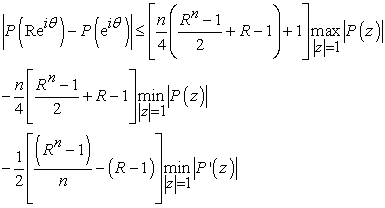from which inequality (4) follows for n > 4. The inequality (5) follows on the same lines as that of inequality (4), but instead of using inequality (6) of Lemma 2, we use the inequality (7) of the same lemma.

### References

  N. C. Ankeny and T. J. Rivlin, On a theorem of S. Bernstein, Pacific J. Math., 5 (1955), 849-852.In article CrossRef  A. Aziz and Q. M. Dawood, Inequalities for a polynomial and its derivative, J. Approx. Theory, 53 (1988), 155-162.In article  K. K. Dewan, Naresh Singh and Abdullah Mir, Growth of polynomials not vanishing inside a circle, Int. Journal of Math. Analysis, 1 (11) (2007), 529-538.In article  M. Riesz, FIU ber einen Satz Herm Serge Bernstein, Acta Math., 40 (1916), 337-343.In article CrossRef